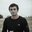Question-and-Answer Resource for the Building Energy Modeling Community
Get s tarted with the Help page

# how to build and minimize the cost function using the output temperature(T) of a thermal system ?if we suppose we have a thermal system for a single Room with two inputs,one for heat pump and the other for the heat of environment and then we Have T(temp) as an output of our system and I want to minimize the cost function having T ,How we should do it (the output)?

Thanks alot.

edit retag close merge delete

Sort by » oldest newest most votedThis question needs to be stated more clearly for your goals to be understood. Typically in a optimization problem you are trying to find a "sweet spot" within a set of model parameters which minimizes (or maximizes) the evaluation of some objective function (sometimes called a cost function).

An example optimization problem might be minimizing annual energy use in the HVAC system by varying the size of some external glazing. Intuitively we hypothesize there may be a "sweet spot" window size because heating energy use can be offset by solar gains, but windows also increase the U-value of the envelope increasing HVAC energy use. So you may set up an optimization problem with the objective function calculating annual HVAC energy use, and the parameter space being the window to wall ratio (WWR).

You wouldn't want to minimize temperature outright in an optimization, this would mean your optimal solution would have the coldest temperature be the optimal solution. Perhaps you want to minimize the time where some temperature range isn't maintained within the zone, where the objective function returns "unmet hours"?

For a clear mathematically rigorous explanation and classification of optimization problems, specifically related to building performance simulation, you should check out the GenOpt Manual, section 4.

more

Thanks for the respond, sorry for the brevity I should have explained it in detail, the Idea is that we want to use split range control system that makes the Tout (of room ) vary between Tmin and Tmax, So the problem would be the minimization of the cost function of the system.according to that my professor gave me this Formula : der(J)=(Tout - ((Tmax+Tmin)/2))^2 but I don't get the idea, is it Ok to minimize the derivative of cost function ?

No you wouldn't want to minimize the derivative of the cost function. Think back to introductory calculus, the derivative is a measure of how much the function is changing. You can find the minimum of a cost function by setting the derivative equal to zero (at minimums and maximum the derivative equals zero) then solve that equation for the variable of interest. For 1D this is easy, for nD (usual BPS) this is hard to visualize.

I would go ask your professor for an overview of the problem. Maybe he can offer you some extra pointers.

thanks for your respond it was a miss understanding from my side.I got it.